Next: Van der Waals Gas Up: Classical Thermodynamics Previous: General Relation Between Specific

# Free Expansion of Gas

Consider a rigid container that is thermally insulated. The container is divided into two compartments separated by a valve that is initially closed. One compartment, of volume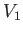, contains the gas under investigation. The other compartment is empty. The initial temperature of the system is. The valve is now opened, and the gas is free to expand so as to fill the entire container, whose volume is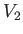. What is the temperature,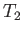, of the gas after the final equilibrium state has been reached?

Because the system consisting of the gas and the container is adiabatically insulated, no heat flows into the system: that is,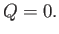(6.144)

Furthermore, the system does no work in the expansion process: that is,(6.145)

It follows from the first law of thermodynamics that the total energy of the system is conserved: that is,(6.146)

Let us assume that the container itself has negligible heat capacity (which, it turns out, is not a particularly realistic assumption--see later), so that the internal energy of the container does not change. Under these circumstances, the energy change of the system is equivalent to that of the gas. The conservation of energy, (6.146), thus reduces to(6.147)

where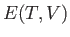is the gas's internal energy.

To predict the outcome of the experiment, it is only necessary to know the internal energy of the gas,, as a function of the temperature and the volume. If the initial parameters,and, are known, as well as the final volume,, then Equation (6.147) yields an equation that specifies the unknown final temperature,.

For an ideal gas, the internal energy is independent of the volume: that is,. (See Section 6.2.) In this case, Equation (6.147) yields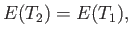(6.148)

which implies that. In other words, there is no temperature change in the free expansion of an ideal gas.

The change in temperature of an non-ideal gas that undergoes free expansion can be written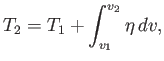(6.149)

where(6.150)

is termed the Joule coefficient. Here,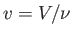is the molar volume, whereis the number of moles of gas in the container. Furthermore,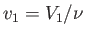and.

According to the first law of thermodynamics,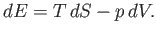(6.151)

However, if the internal energy is constant then we can write(6.152)

With the aid of Equations (6.109) and (6.120), this becomes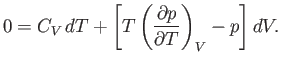(6.153)

Hence,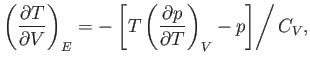(6.154)

and the Joule coefficient can be written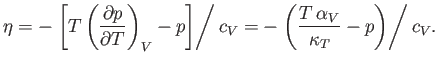(6.155)

[See Equation (6.132).] Note that all terms on the right-hand side of the previous expression are easily measurable.Next: Van der Waals Gas Up: Classical Thermodynamics Previous: General Relation Between Specific
Richard Fitzpatrick 2016-01-25